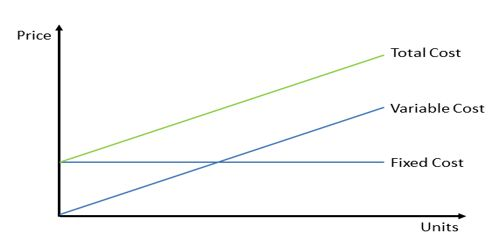# “Variable costs are always Relevant Cost” – Explanation

Variable costs are always a relevant cost: Variable costs are relevant costs only if they differ in total between the alternatives under consideration. Variable costs are always relevant cost, I agree with this conception. Because, I know that, the Variable cost is a cost that charges of resources and activity direct proportion in charges in the output measure of resources and activities. And Relevant Cost is the limit of output measure of resources and activity within which a specific relationship between cost and output measure of resources and activity is valid. Relevant costs are those costs that differ among the alternative courses of action. In some situations (such as make or buy) most variable costs would be considered relevant. However, there are many situations when some or all variable costs would be the same for two alternatives and therefore not be relevant.

Now, I can say that a relevant cost also applies to variable costs. That is, outside a relevant cost, some variable cost, such a fuel consumed, may behave differently per unit of output measure of resources and activity. For example, the variable cost of a canning machine at Del Monte might be \$5 for every hour it is used, assuming that it will be used between 30-50 hours each week. However, if it is used for more than 50 hours a week, the added were and tear might increase variable costs to \$ 6 for those hours beyond 50. So, “Variable casts always relevant cost”.